# Expanded Computational Complexity Graph

WWW Graph of Computational Complexity for Link Analysis Ranking Experiments

WWW Graph of Computational Complexity for Link Analysis Ranking Experiments: The Base Set is constructed by including all out-links of each page in the Root Set. We use the refined algorithm for detecting intra-domain links.

(884 vertices, 1616 edges)

## Examples

### Basic Examples

Retrieve the graph:

 In:=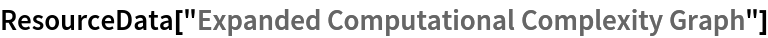Out=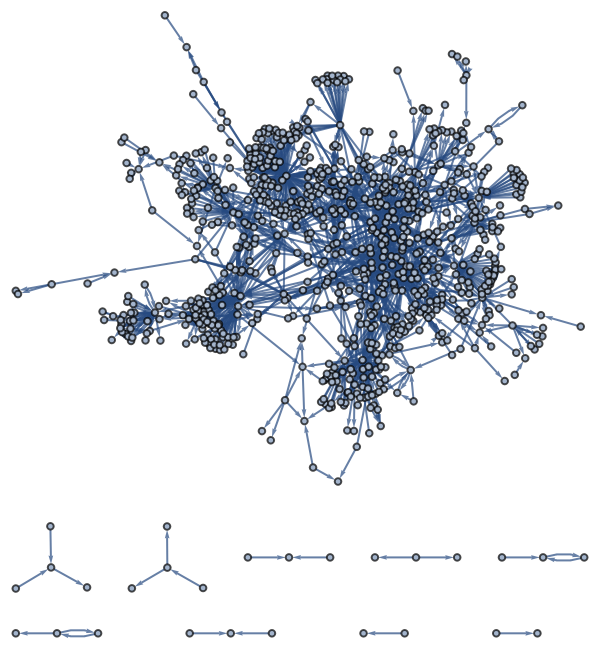Summary properties:

 In:=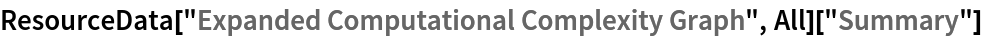Out=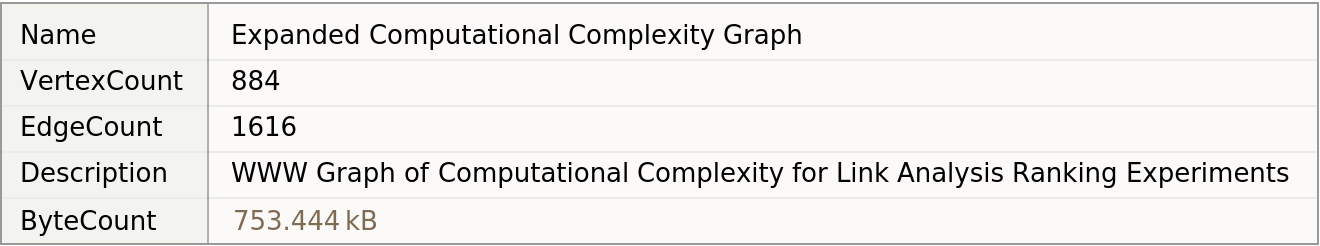### Basic Applications

Show the power-law degree distribution of the graph:

 In:=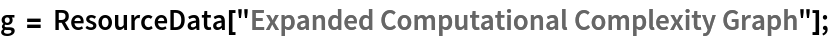In:=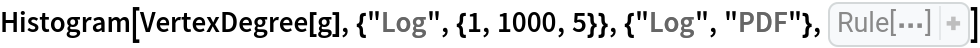Out=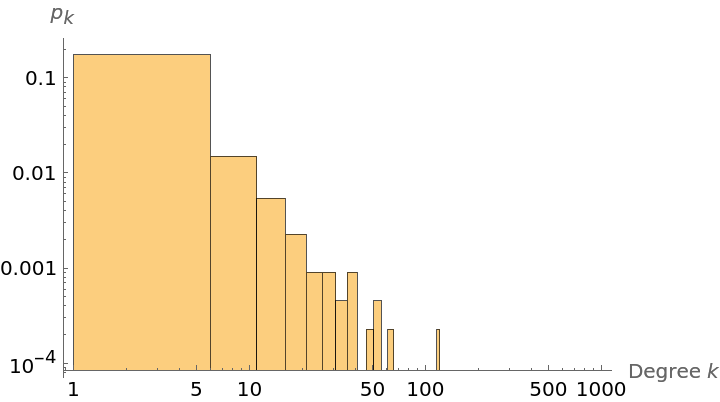Show a table of properties:

 In:=Out=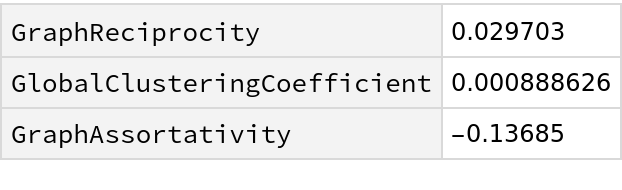Wolfram Research, "Expanded Computational Complexity Graph" from the Wolfram Data Repository (2019)